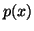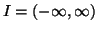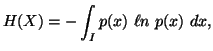Next:Differential Entropy and ProbabilityUp:Shannon's Entropy

Entropy of a Continuous Random Variable

Shannon's entropy though defined for a discrete random variable can be extended to situations when the random variable under consideration is continuous.

Let X be a continuous random variable with probability density functionon I, where, then the entropy is given by(1.13)

whenever it exists. The measure (1.13) sometimes called "differential entropy". It has many of the properties of discrete entropy but unlike the entropy of a discrete random variable that of a continuous random variable may be infinitely large, negative or positive (Ash, 1965 ). The entropy of a discrete random variable remains invariant under a change of variable, however with a continuous random variable the entropy does not necessarily remain invariant.

Subsections

21-06-2001
Inder Jeet Taneja
Departamento de Matemática - UFSC
88.040-900 Florianópolis, SC - Brazil